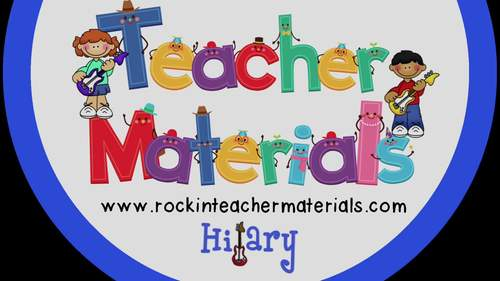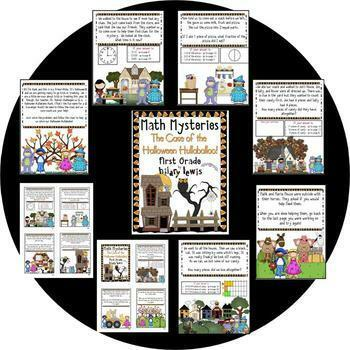# 1st Grade Word Problems - Math Mystery - Case of the Halloween HullabalooResource Type
File Type
PDF (37 MB|27 pages)
Standards
\$5.99
List Price:
\$6.99
You Save:
\$1.00
• Product Description
• Standards

This is a fun way for 1st graders to practice solving word problems in a unique way. Kids start reading at the beginning of the book, solve the problems, and go where the book tells them to go! If they get the wrong answer, they will have a 'stopper' that will tell them to go back to where they were working, and TRY AGAIN!

The number of words in this story have been reduced and simplified for our first grade friends. You can read together in groups, as a class or kids can read them individually.

This mystery includes these skills:

*time to the hour

*simple fractions

*numbers and number words

*shapes

*halves

*smallest to largest

*even and odd numbers

*tally marks

There are a total of 10 word problems included in this story.

I use these mysteries at my math stations during guided math. I am sure your kids will love this Halloween themed math mystery book as much as mine do! What the next mystery will bring?

Check Out my Other Math Mysteries!

Thanks!

Hilary

Check out my blog!

Look for and express regularity in repeated reasoning. Mathematically proficient students notice if calculations are repeated, and look both for general methods and for shortcuts. Upper elementary students might notice when dividing 25 by 11 that they are repeating the same calculations over and over again, and conclude they have a repeating decimal. By paying attention to the calculation of slope as they repeatedly check whether points are on the line through (1, 2) with slope 3, middle school students might abstract the equation (𝑦 – 2)/(𝑥 – 1) = 3. Noticing the regularity in the way terms cancel when expanding (𝑥 – 1)(𝑥 + 1), (𝑥 – 1)(𝑥² + 𝑥 + 1), and (𝑥 – 1)(𝑥³ + 𝑥² + 𝑥 + 1) might lead them to the general formula for the sum of a geometric series. As they work to solve a problem, mathematically proficient students maintain oversight of the process, while attending to the details. They continually evaluate the reasonableness of their intermediate results.
Look for and make use of structure. Mathematically proficient students look closely to discern a pattern or structure. Young students, for example, might notice that three and seven more is the same amount as seven and three more, or they may sort a collection of shapes according to how many sides the shapes have. Later, students will see 7 × 8 equals the well remembered 7 × 5 + 7 × 3, in preparation for learning about the distributive property. In the expression 𝑥² + 9𝑥 + 14, older students can see the 14 as 2 × 7 and the 9 as 2 + 7. They recognize the significance of an existing line in a geometric figure and can use the strategy of drawing an auxiliary line for solving problems. They also can step back for an overview and shift perspective. They can see complicated things, such as some algebraic expressions, as single objects or as being composed of several objects. For example, they can see 5 – 3(𝑥 – 𝑦)² as 5 minus a positive number times a square and use that to realize that its value cannot be more than 5 for any real numbers 𝑥 and 𝑦.
Use appropriate tools strategically. Mathematically proficient students consider the available tools when solving a mathematical problem. These tools might include pencil and paper, concrete models, a ruler, a protractor, a calculator, a spreadsheet, a computer algebra system, a statistical package, or dynamic geometry software. Proficient students are sufficiently familiar with tools appropriate for their grade or course to make sound decisions about when each of these tools might be helpful, recognizing both the insight to be gained and their limitations. For example, mathematically proficient high school students analyze graphs of functions and solutions generated using a graphing calculator. They detect possible errors by strategically using estimation and other mathematical knowledge. When making mathematical models, they know that technology can enable them to visualize the results of varying assumptions, explore consequences, and compare predictions with data. Mathematically proficient students at various grade levels are able to identify relevant external mathematical resources, such as digital content located on a website, and use them to pose or solve problems. They are able to use technological tools to explore and deepen their understanding of concepts.
Model with mathematics. Mathematically proficient students can apply the mathematics they know to solve problems arising in everyday life, society, and the workplace. In early grades, this might be as simple as writing an addition equation to describe a situation. In middle grades, a student might apply proportional reasoning to plan a school event or analyze a problem in the community. By high school, a student might use geometry to solve a design problem or use a function to describe how one quantity of interest depends on another. Mathematically proficient students who can apply what they know are comfortable making assumptions and approximations to simplify a complicated situation, realizing that these may need revision later. They are able to identify important quantities in a practical situation and map their relationships using such tools as diagrams, two-way tables, graphs, flowcharts and formulas. They can analyze those relationships mathematically to draw conclusions. They routinely interpret their mathematical results in the context of the situation and reflect on whether the results make sense, possibly improving the model if it has not served its purpose.
Reason abstractly and quantitatively. Mathematically proficient students make sense of quantities and their relationships in problem situations. They bring two complementary abilities to bear on problems involving quantitative relationships: the ability to decontextualize-to abstract a given situation and represent it symbolically and manipulate the representing symbols as if they have a life of their own, without necessarily attending to their referents-and the ability to contextualize, to pause as needed during the manipulation process in order to probe into the referents for the symbols involved. Quantitative reasoning entails habits of creating a coherent representation of the problem at hand; considering the units involved; attending to the meaning of quantities, not just how to compute them; and knowing and flexibly using different properties of operations and objects.
Total Pages
27 pages